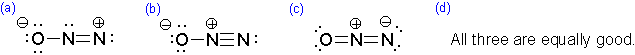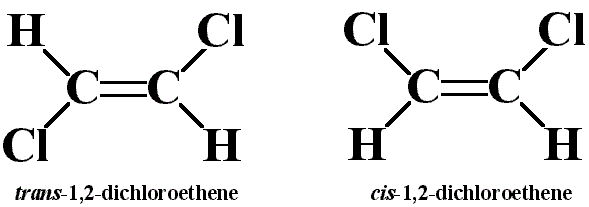# Exercise 9

$$\newcommand{\vecs}{\overset { \rightharpoonup} {\mathbf{#1}} }$$ $$\newcommand{\vecd}{\overset{-\!-\!\rightharpoonup}{\vphantom{a}\smash {#1}}}$$$$\newcommand{\id}{\mathrm{id}}$$ $$\newcommand{\Span}{\mathrm{span}}$$ $$\newcommand{\kernel}{\mathrm{null}\,}$$ $$\newcommand{\range}{\mathrm{range}\,}$$ $$\newcommand{\RealPart}{\mathrm{Re}}$$ $$\newcommand{\ImaginaryPart}{\mathrm{Im}}$$ $$\newcommand{\Argument}{\mathrm{Arg}}$$ $$\newcommand{\norm}{\| #1 \|}$$ $$\newcommand{\inner}{\langle #1, #2 \rangle}$$ $$\newcommand{\Span}{\mathrm{span}}$$ $$\newcommand{\id}{\mathrm{id}}$$ $$\newcommand{\Span}{\mathrm{span}}$$ $$\newcommand{\kernel}{\mathrm{null}\,}$$ $$\newcommand{\range}{\mathrm{range}\,}$$ $$\newcommand{\RealPart}{\mathrm{Re}}$$ $$\newcommand{\ImaginaryPart}{\mathrm{Im}}$$ $$\newcommand{\Argument}{\mathrm{Arg}}$$ $$\newcommand{\norm}{\| #1 \|}$$ $$\newcommand{\inner}{\langle #1, #2 \rangle}$$ $$\newcommand{\Span}{\mathrm{span}}$$

Solutions, hints and answers can be found in the Solutions Page. It is strongly recommended that students do not look at the solutions until after completing or attempting to complete the problems. Going straight to the solutions will reduce the point of the exercises.

## Q1

The central atom in $$BrF_5$$ has how many bonding pairs of electrons and how many non-bonding pairs of electrons.

## Q2

4. Which of the following molecules does not have a net dipole moment?

1. $$H_2O$$
2. $$NH_3$$
3. $$BF_3$$
4. $$BrF_5$$

## Q3

What is the bond order in $$NO_2^+$$?

1. 1.5
2. 2
3. 3
4. 4

## Q4

Which of the following best represents the 3-dimensional view of $$H_2N^-$$?## Q5

Which of the following represents the best resonance structure for $$N_2O$$?## Q6

Draw acceptable Lewis structures for the following molecules: $$BH_4^-$$, $$NF_3$$, $$CH_3OH$$, $$CO$$, $$H_2CO_3$$ (the hydrogen atoms are bonded to oxygen atoms), $$H_2O_2$$, $$HOF$$, $$H_2CO$$.

## Q7

The remarkable reactivity of fluorine enables the synthesis of an unusually diverse array of compounds of fluorine and iodine. The set includes $$IF$$, $$IF_2^+$$, $$IF_4^+$$, $$IF_4^-$$, $$IF_5$$, and $$IF_8^-$$. Draw the electron-dot (valence-bond) structures of each species and predict its structure.

## Q8

Consider the cis and trans isomers for 1,2-dichloroethene ($$C_2H_2Cl_2$$):Identify the differences in:

1. molecular mass
2. dipole moments
3. Lewis structures
4. molecular geometries

## Q9

Iodine reacts with fluorine to yield several compounds with the molecular formula $$IF_n$$ with $$n$$ varying from 1, 3, 5, and 7.

1. Draw the Lew structure of each.
2. Draw the three-dimensional structure of each molecule with $$n<7$$.

## Q10

Draw acceptable Lewis structure(s) for the chlorite anion, $$ClO_2^-$$. What consequences does the Lewis structure(s) have for the bond angle and bond lengths in this anion? What are the bond order(s) of the $$\ce{Cl-O}$$ bonds?

## Q11

Two isomers with the stoichiometry $$CH_3NO_2$$ are known: methyl nitrite with the connectivity $$H_3CONO$$ and nitromethane with the connectivity $$H_3CNO_2$$.

1. Draw the Lewis structures of the two isomers. Include resonance structures as appropriate. Provide the formal charges of the heavy, i.e. non-hydrogen, atoms.
2. Compare the nitrogen-oxygen bond lengths of the two isomers. Discuss the basis for your order.
3. Predict the $$CON$$ and $$ONO$$ bond angles in methyl nitrite.
4. In which isomer would you expect all the heavy atoms to lie in the same plane?

## Q12

Fill in the following tables (follows octet rules):

 Species # of electron groups VB hybridization number of atoms bonded to central atom electronic geometry name molecular geometry name bond angles Polar or nonpolar $$CS_2$$ $$SO_3$$ $$SO_3^{2-}$$ $$NH_3$$ $$SF_6$$ $$O_3$$ $$SF_4$$ $$XeF_4$$ $$PO_4^{3-}$$ $$CH_2Cl_2$$

## Q13

Use the information in the table of Q12 to draw each of the (3-D) molecules.

## Q14

Write resonance structures for the nitrate ion ($$NO_3^-$$), if any exist. What are the bond orders of the bonds involved in this ion?

Exercise 9 is shared under a not declared license and was authored, remixed, and/or curated by LibreTexts.